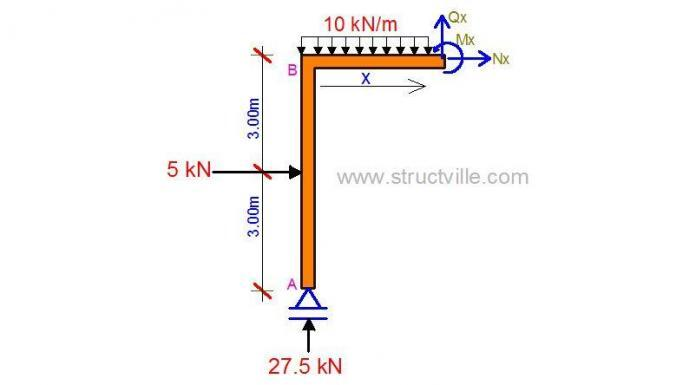# Question of the day | 22-03-2021For the section of a frame cut as shown in the figure above;

1. Generate the equation for bending moment along the beam.
(A) Mx = 5x + 27.5 – 10x2
(B) Mx = 27.5x – 5x2 – 15
(C) Mx = 27.5x – 10x2 + 15
(D) Mx = 5x + 27.5x2 + 10x

2. Generate the equation for shear force on the beam.
(A) Qx = 5x + 27.5 – 10x
(B) Qx = 27.5x + 10x
(C) Qx = 5x – 10
(D) Qx = 27.5 – 10x

3. What the axial force on column AB?
(A) 27.5 kN (compression)
(B) 10 kN (Tension)
(C) 5 kN (Compression)
(D) 17.5 kN (Tension)

1.AneeS

Nice

2.Ricardo Vale Pereira

B;D;A

3.Sebastian D Urciullo

Hi, where do I check my answers to the daily questions ?

4.Stefanos K

B, D, A